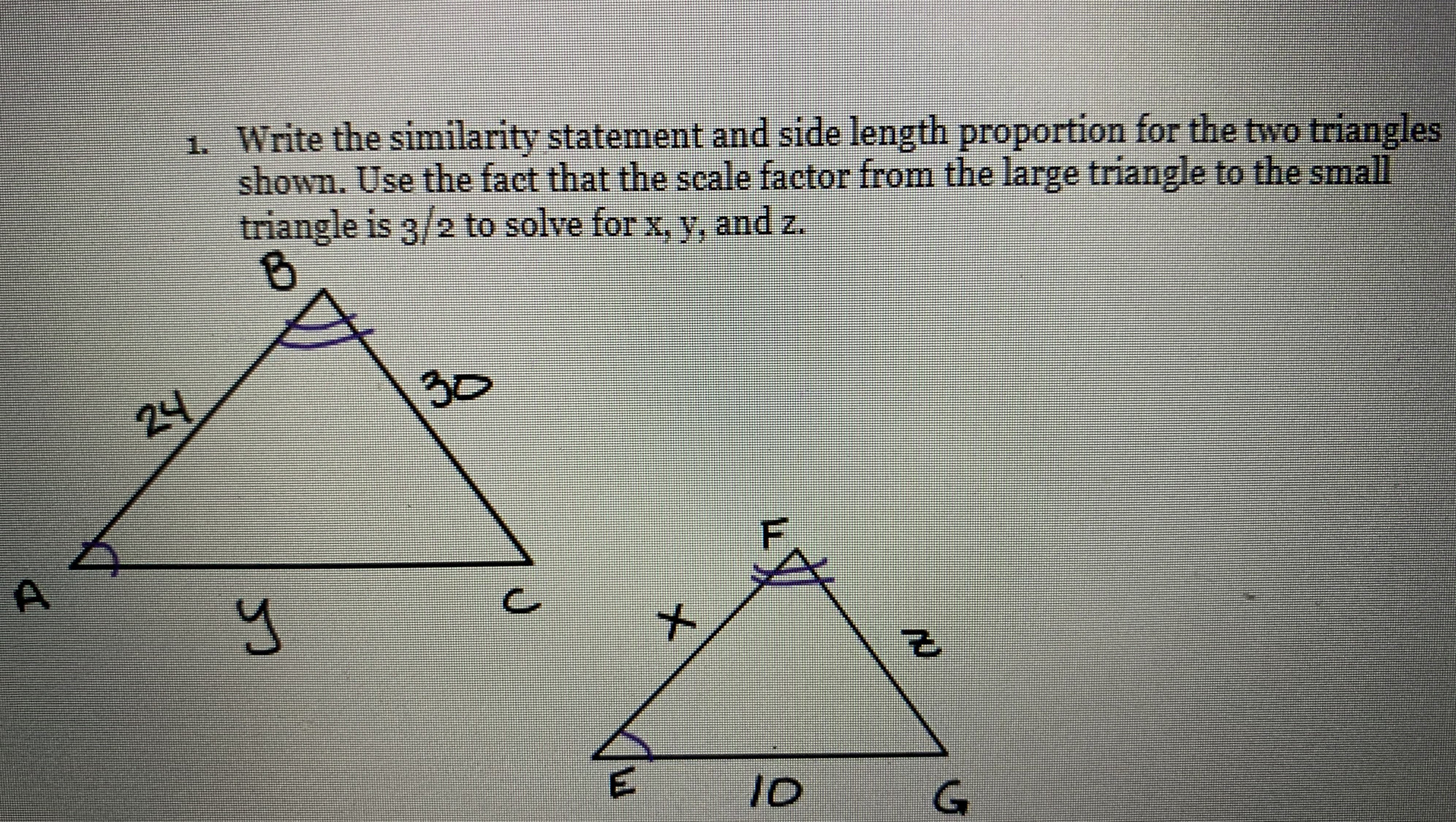Still have math questions?

Geometry
QuestionWrite the similarity statement and side length proportion for the two triangles shown. Use the fact that the scale factor from the large triangle to the small triangle is 3/2 to solve for $$x$$$$y$$, and $$z$$.

$$x=16,~y=15,~z=20$$